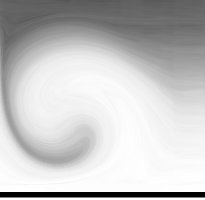## She's the 1

zur Melodie von "She's the one" von Robbie Williams

```"I" was her	back in Rome
But she's 1	here at home
And if there's a number
Times n is n,
She's the 1.
If there's a number
Times n is n,
She's the 1.

She and oh's	all you need
to work in	binary
And if there's a number
Times n is n,
She's the 1.
If there's a number
Times n is n,
She's the 1.

When you take some to what you got
And want to know what's in the set
When you write her left on the row
And then one thousand times "zero"
That'll be to hight, that's mind-boggling

Facutly 	might be strong
But 1 !		that's just 1
And if there's a number
Times n is n,
She's the 1.
If there's a number
Times n is n,
She's the 1.

When you take some to what you got
And want to know what's in the set
When you write her left on the row
And then one thousand times 0
That'll be to hight, that's mind-boggling

"I" was her	back in Rome
But she's 1	here at home
And if there's a number
Times n is n,
She's the 1.
If there's a number
Times n is n,
She's the 1.

If there's a number
Times n is n,
She's the 1.
Yeah she's the 1

If there's a number
Times n is n,
She's the 1.
She's the 1.

If there's a number
Times n is n,
She's the 1.

Singhinweise:
!: "bang"
```
Go up